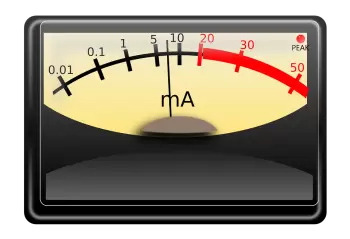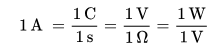# What Is One Ampere? Definition of AmpThe ampere is the base unit of electric current in the international system of units (SI) and is expressed with the symbol (A). Its magnitude corresponds to the amount of electric charge that crosses a section of an electric conductor.

This unit of measurement takes its name from the French physicist and mathematician André-Marie Ampère (1775-1836), one of the leading scholars of electromagnetism.

All other electromagnetic units are derived from one of the seven SI base units.

The electric charge, the number of elementary charges (electrons), can be expressed in ampere-hours (Ah). The ampere-hour is the total amount of charge that flows in a conductor with the intensity of one amperage in one hour.

To measure electrical flow, we can use a device called an ammeter.

## What Is the Ampere Definition (amp)?

There are two definitions of ampere:

### 1. Definition of Ampere Based on Electrical Phenomena

An ampere is the intensity of the electric current that passes through a conductor in a section (circular or not). One ampere is equal to the one-coulomb charge that passes through the area in one second.

By analogy, the intensity of the current in an electrical circuit is comparable to the amount of water that passes through a tube measured in kg/second, where the mass of water represents the amount of electrical energy.

### 2. Definition of Ampere Based on Magnetic Phenomena

The ampere is that constant current which, if maintained in two straight parallel conductors of infinite length, of negligible circular cross-section, and placed 1 meter apart in vacuum, would produce between these conductors a force equal to 2 x 10-7 newton per meter of length.

According to Ampere's law, the ampere is that constant current flow in two parallel wires of infinite length and placed at a distance of one meter so that they attract each other with force equal to 2 × 10 - 7N/m.

## Ohm's Law and Electrical Power

Within SI units, according to Ohm's law, electric current can be related to the voltage and electrical resistance of an electrical circuit. In addition, together with the law of electric power, the following equality applies:With "C" for coulombs, "s" for seconds, "V" for volts, "Ω" for ohms, and "W" for watts. The absence of coefficients is because the International System is a coherent measurement system.

The amperage of one ampere is the flow of one coulomb per second. At the same time, it equals the voltage of one volt of the charge moving through the resistance of one ohm.

## What Is the Difference Between Volt and Ampere?

The volt is the unit of the international system of measurements for measuring the voltage of an electric current. Ampere is the unit of a global measurement system for measuring current intensity.

Therefore, we should know:

### What Is the Difference Between Voltage and Current Intensity?

The intensity of an electric current is the electric charge (number of electrons) that circulates in a conductor per unit time (per second). On the other hand, the voltage of an electric current is the force with which it does it. This difference is called the potential difference.

### Example:

When comparing it with a water pipe, we can imagine that the electrons are drops of water. In this case, the current intensity is the number of water drops circulating through the pipe. In this simile, pressure is the difference in potential or current voltage.

Author:

Published: September 6, 2018
Last review: August 23, 2022